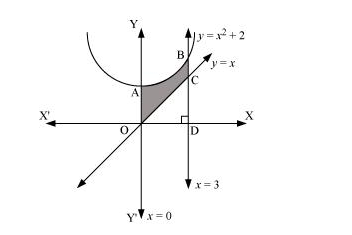# Find the area of the region bounded by the curves

Question:

Find the area of the region bounded by the curves $y=x^{2}+2, y=x, x=0$ and $x=3$

Solution:

The area bounded by the curves, $y=x^{2}+2, y=x, x=0$, and $x=3$, is represented by the shaded area OCBAO asThen, Area OCBAO = Area ODBAO – Area ODCO

$=\int_{0}^{3}\left(x^{2}+2\right) d x-\int_{0}^{3} x d x$

$=\left[\frac{x^{3}}{3}+2 x\right]_{0}^{3}-\left[\frac{x^{2}}{2}\right]_{0}^{3}$

$=[9+6]-\left[\frac{9}{2}\right]$

$=15-\frac{9}{2}$

$=\frac{21}{2}$ units